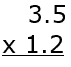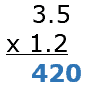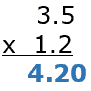# Decimal Multiplication Worksheets

## All About These 15 Worksheets

These Decimal Multiplication worksheets are designed to help students master the concept of multiplying decimal numbers. The worksheets include a variety of creative and engaging exercises to help students build their skills and confidence in decimal multiplication.

Each worksheet in the series includes a mix of problems with varying degrees of difficulty, designed to challenge and engage students at different skill levels. The problems typically require students to multiply decimal numbers with different numbers of decimal places and to properly place the decimal point in the product.

These worksheets also provide ample space for students to show their work and write down their answers. This helps them develop their problem-solving skills and mental math abilities.

This series of worksheets is most suitable for students in grades 5 to 7. It can be used in the classroom as a supplement to the math curriculum or at home as a way for parents to help their children practice and reinforce their math skills.

Overall, these Decimal Multiplication worksheets provide a comprehensive and engaging way for students to develop their skills and confidence in multiplying decimal numbers. With these worksheets, students can build a solid foundation in math and develop the skills they need to succeed in higher-level math courses.

## How to Multiply Decimal Values

Multiplying decimal values is similar to multiplying whole numbers, with an additional step to account for the decimal points. Here’s a step-by-step guide to multiplying decimal values that teachers can share with their students:

• Ignore the Decimal Points Temporarily – Treat both decimal numbers as if they were whole numbers. This will make the multiplication process easier.
• Multiply the Numbers – Use standard multiplication techniques to multiply the numbers, as if they were whole numbers.
• Count the Total Number of Decimal Places – Add up the total number of decimal places in both of the original decimal numbers. This will determine the position of the decimal point in the product.
• Place the Decimal Point – Starting from the rightmost digit of the product, move the decimal point to the left the same number of places as the total number of decimal places counted in step 3.
• Simplify, if necessary – If the product has trailing zeros after the decimal point (e.g., 4.500), you can remove them to simplify the result (e.g., 4.5).

Here’s an example of multiplying 1.2 and 3.5:

Step 1: Ignore the Decimal Points Temporarily – Treat 1.2 as 12 and 3.5 as 35.Step 2: Multiply the Numbers – Multiply 12 and 35, which equals 420.Step 3: Count the Total Number of Decimal Places – There is one decimal place in 1.2 and one in 3.5, so there are a total of 2 decimal places.

Step 4: Place the Decimal Point – Starting from the rightmost digit of the product (420), move the decimal point 2 places to the left, resulting in 4.20.Step 5: Simplify, if necessary – Remove the trailing zero to get 4.2.

The product of 1.2 and 3.5 is 4.2.

## How to Teach Students to Multiply Decimals

Teaching students to multiply decimals involves breaking down the process into manageable steps and using various teaching methods to reinforce understanding. Here’s a suggested approach for teaching students how to multiply decimals:

• Review basic multiplication – Before teaching decimal multiplication, ensure students have a strong foundation in basic multiplication with whole numbers.
• Reinforce decimal understanding – Review decimal place values (tenths, hundredths, etc.) and ensure students have a solid grasp of decimals before introducing multiplication.
• Ignore the decimal points initially – Teach students to first multiply the numbers as if they were whole numbers. This simplifies the process and allows them to focus on the multiplication itself.
• Count decimal places – Instruct students to count the total number of decimal places in both numbers being multiplied. This is essential for placing the decimal point in the final answer.
• Place the decimal point – After multiplying the numbers as whole numbers, students should place the decimal point in the final answer, making sure the total number of decimal places in the answer matches the combined total from both original numbers.
• Practice with examples – Provide students with a variety of examples of decimal multiplication problems. Start with simpler problems and gradually increase the difficulty as they become more comfortable with the process.
• Use visual aids and manipulatives – To help students better understand decimal multiplication, use visual aids like grid paper or manipulatives such as base-ten blocks. These tools can help students visualize the multiplication process and better comprehend the concept.
• Real-life applications – Incorporate real-life scenarios where decimal multiplication is required, such as calculating areas, prices, or measurements. This helps students see the practical applications of their new skill and reinforces learning.
• Connect with fractions – Show students the relationship between decimals and fractions, and teach them how to convert decimals to fractions and vice versa. This can help deepen their understanding of decimals and provide another method for solving multiplication problems.
• Review and practice regularly – Encourage students to review and practice decimal multiplication consistently to build their confidence and fluency.

By using this step-by-step approach and incorporating a variety of teaching methods, students can effectively learn to multiply decimals and develop a strong foundation in this essential mathematical skill. Remember to be patient and provide support as needed, adjusting the teaching approach to suit individual learning styles and needs.